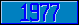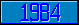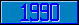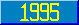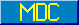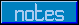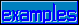## MWAPI - Errors

### Meaning of M? codes in 1995 standard

In some renditions of the 1995 standard, the error message codes appear as "M?nnn". The meaning of these numbers is:

 M?1 = M46 M?2 = M47 M?3 = M48 M?4 = M49 M?5 = M50 M?6 = M51 M?7 = M52 M?8 = M53 M?9 = M54 M?10 = M55